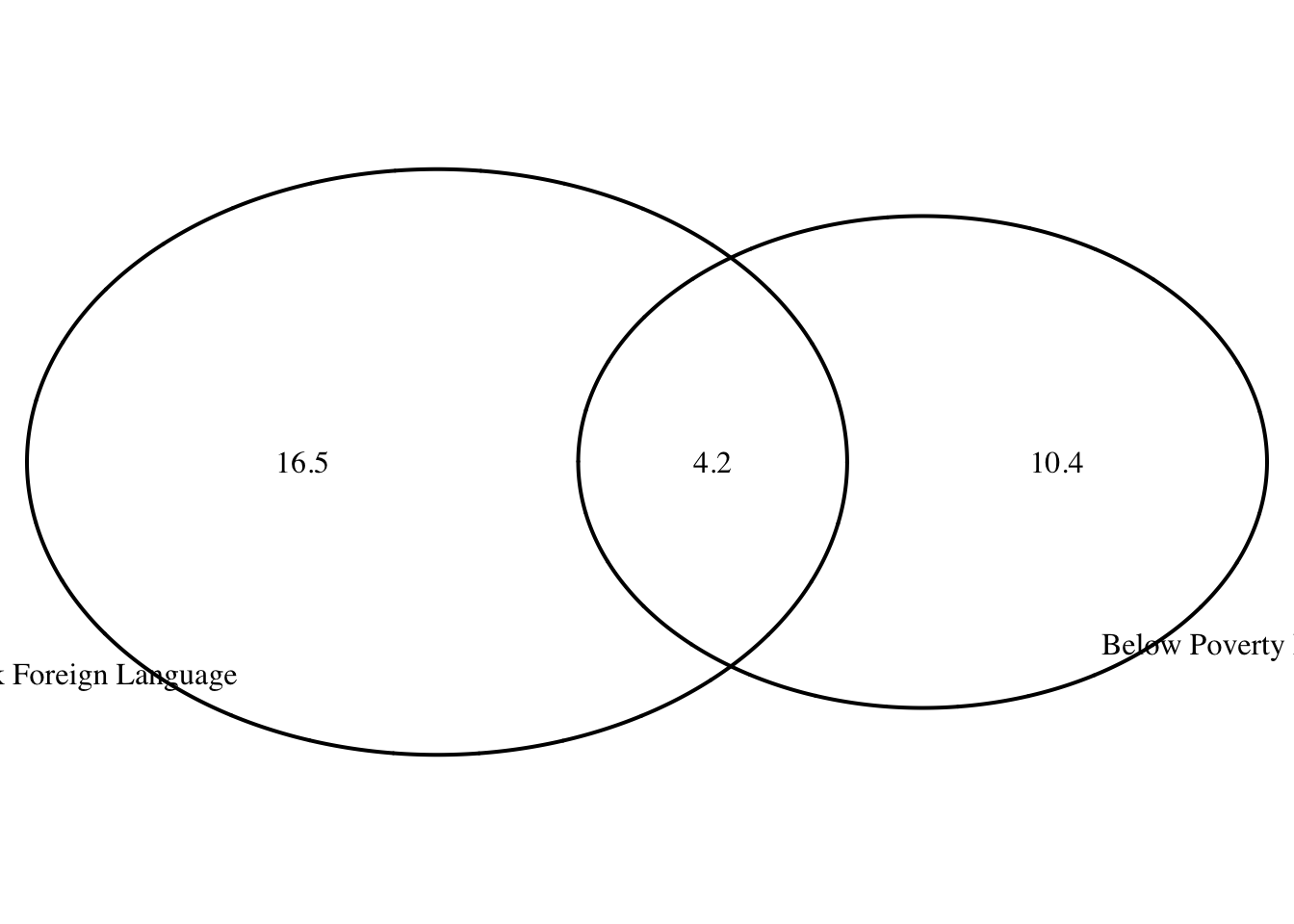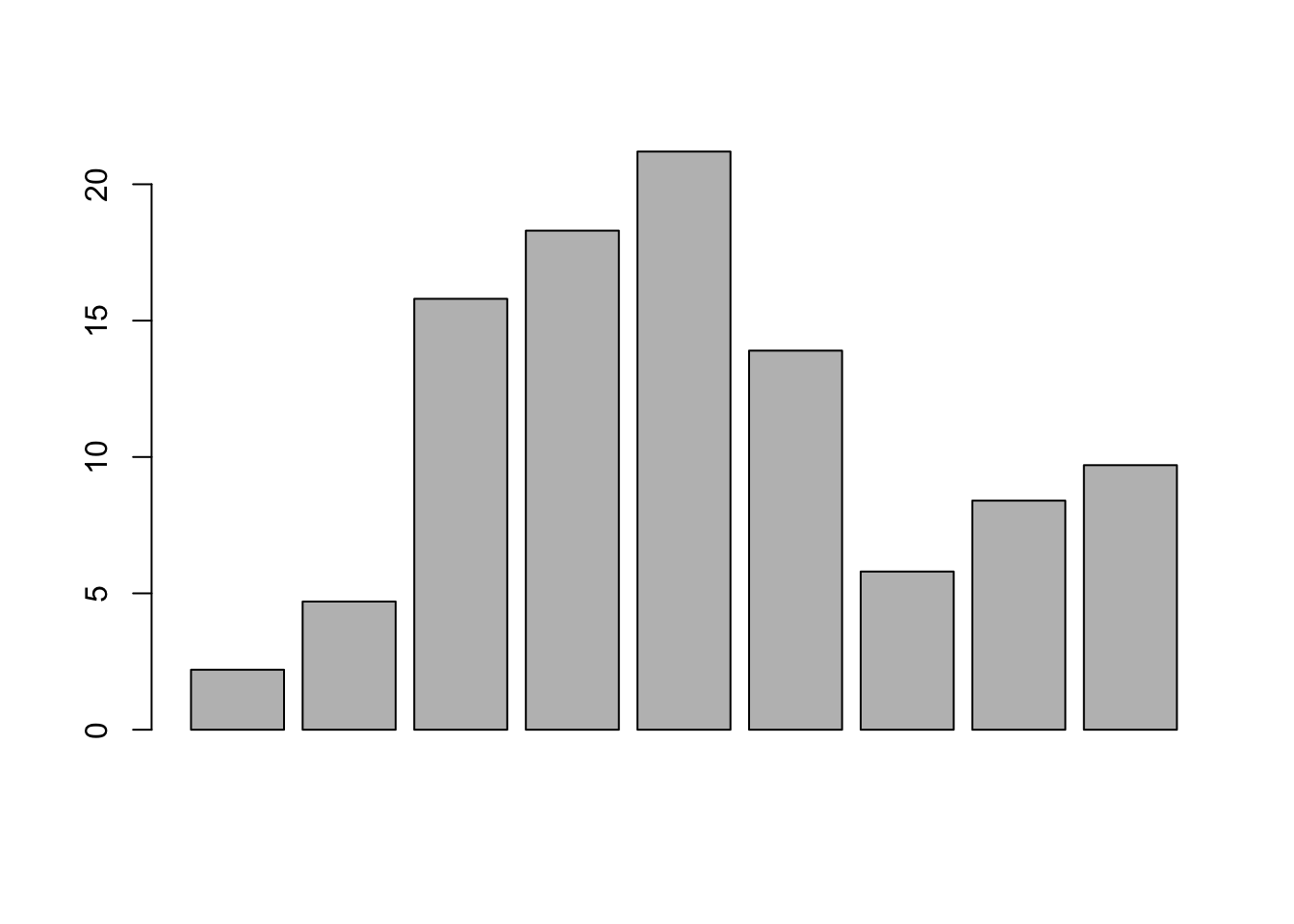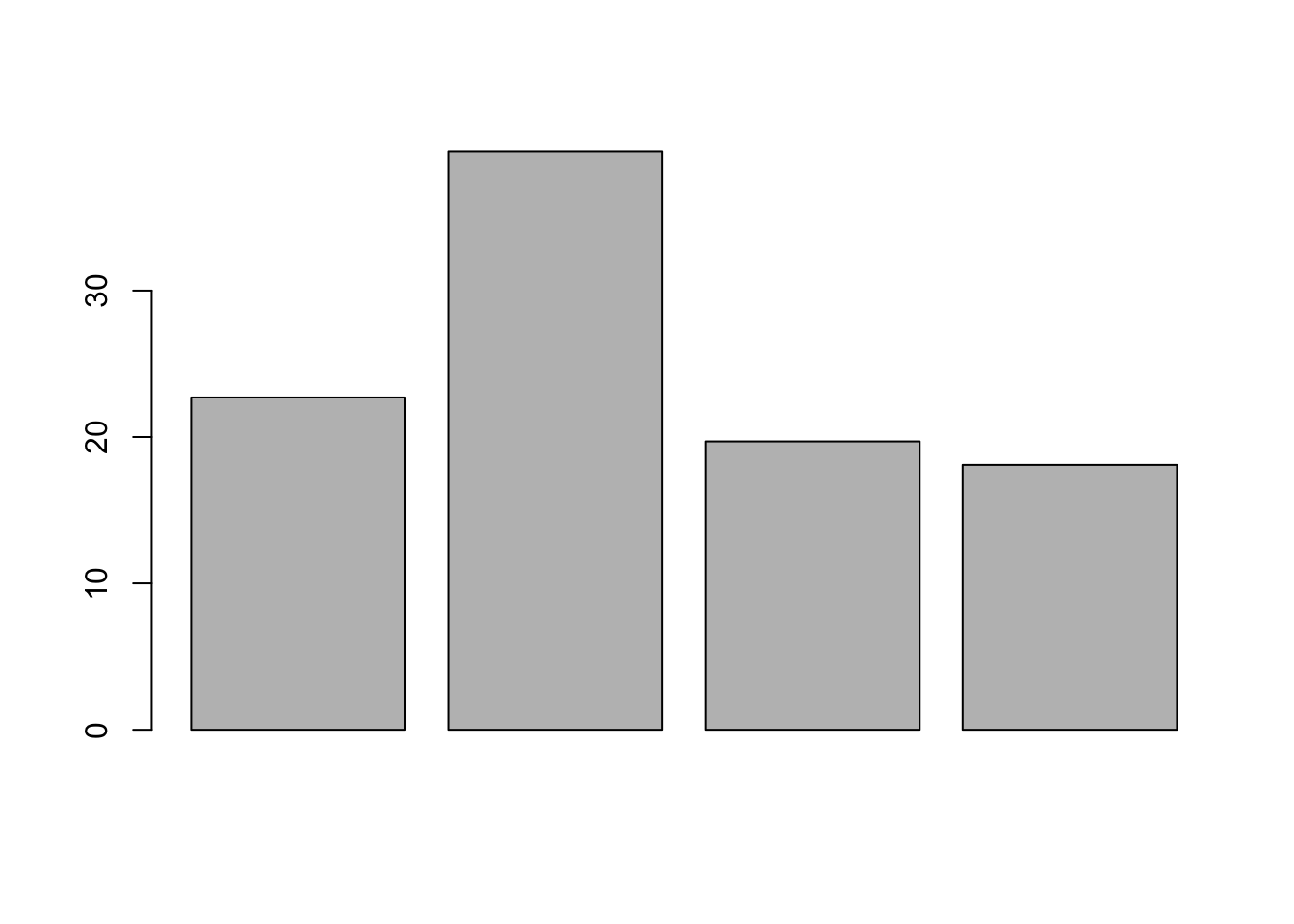#### Elina Azrilyan

##### 09/18/2018

Chapter 2 - Probability Practice: 2.5, 2.7, 2.19, 2.29, 2.43 Graded: 2.6, 2.8, 2.20, 2.30, 2.38, 2.44

2.6 Dice rolls. If you roll a pair of fair dice, what is the probability of

1. getting a sum of 1?

Probability is 0 - there is no way of getting a sum of 1 when we roll 2 dice. The sum will be at least 2.

1. getting a sum of 5?

There are 12 possible ways a roll of 2 dice can turn out. How many of these combinations return as sum of 5: - 1 and 4 - 4 and 1 - 2 and 3 - 3 and 2

Probability of each of these is 1/6 * 1/6 = 1/36. We add those to get 4/36. The answer is 1/9.

1. getting a sum of 12?

The only way of getting a sum of 12 is rolling a 6 and another 6. So the probability of that is 1/6 * 1/6 = 1/36.

2.8 Poverty and language. The American Community Survey is an ongoing survey that provides data every year to give communities the current information they need to plan investments and services. The 2010 American Community Survey estimates that 14.6% of Americans live below the poverty line, 20.7% speak a language other than English (foreign language) at home, and 4.2% fall into both categories. (a) Are living below the poverty line and speaking a foreign language at home disjoint?

No those two are not disjoint.

1. Draw a Venn diagram summarizing the variables and their associated probabilities.
#install.packages('VennDiagram')
library(VennDiagram)
grid.newpage()
draw.pairwise.venn(area1 = 14.6, area2 = 20.7, cross.area = 4.2, category = c("Below Poverty Line", "Speak Foreign Language"))## (polygon[GRID.polygon.1], polygon[GRID.polygon.2], polygon[GRID.polygon.3], polygon[GRID.polygon.4], text[GRID.text.5], text[GRID.text.6], text[GRID.text.7], text[GRID.text.8], text[GRID.text.9])
1. What percent of Americans live below the poverty line and only speak English at home?

10.4%

1. What percent of Americans live below the poverty line or speak a foreign language at home?

20.7+14.6-4.2 = 31.1

1. What percent of Americans live above the poverty line and only speak English at home?

100-14.6 = 85.4 <- Americans above poverty line

85.4-16.5 = 68.9 <- subtracting those over poverty like for speak a goreign language

1. Is the event that someone lives below the poverty line independent of the event that the person speaks a foreign language at home?

If P(A occurs, given that B is true) = P(A | B) = P(A), then A and B are independent.

P(A|B) = 4.2%

P(A) = 14.6%

The events are not independent.

2.20 Assortative mating. Assortative mating is a nonrandom mating pattern where individuals with similar genotypes and/or phenotypes mate with one another more frequently than what would be expected under a random mating pattern. Researchers studying this topic collected data on eye colors of 204 Scandinavian men and their female partners. The table below summarizes the results. For simplicity, we only include heterosexual relationships in this exercise.65

1. What is the probability that a randomly chosen male respondent or his partner has blue eyes?

P(A|B) = P(A)+P(B)-P(A and B) = 114/204+108/204-78/204 = 144/204 = 0.71

1. What is the probability that a randomly chosen male respondent with blue eyes has a partner with blue eyes?

78/114 = 0.68

1. What is the probability that a randomly chosen male respondent with brown eyes has a partner with blue eyes?

19/54 = 0.35

What about the probability of a randomly chosen male respondent with green eyes having a partner with blue eyes?

11/36 = 0.31

1. Does it appear that the eye colors of male respondents and their partners are independent? Explain your reasoning.

It appears that the eye color of male respondents and their partners is dependent, since P(partner having blue eyes) varies by male eye color as evident from our calculations above.

2.30 Books on a bookshelf. The table below shows the distribution of books on a bookcase based on whether they are nonfiction or fiction and hardcover or paperback.

1. Find the probability of drawing a hardcover book first then a paperback fiction book second when drawing without replacement.

P(Hardcover first) = 28/95

P(Paperback Fiction 2nd) = 59/94

P = (28/95) * (59/94) = 0.295 * 0.628 = 0.185

1. Determine the probability of drawing a fiction book first and then a hardcover book second, when drawing without replacement.

P(Fiction 1st) = 72/95 = 0.758

Scenario 1: 1st fiction drawn was a hardcover

P (Hardcover 2nd) = 27/94 = 0.287

P (Hardcover given that it is Fiction) = 13/72 = 0.181

Scenario 2: 1st fiction book drawn was paperback

P(Hardcover 2nd) = 28/94 = 0.298

P (Paperback given that it is Ficiton) = 1-0.181 = 0.819

P = (0.7580.287 0.181)+(0.758 0.298 0.819)=0.039+0.185=0.224

1. Calculate the probability of the scenario in part (b), except this time complete the calculations under the scenario where the first book is placed back on the bookcase before randomly drawing the second book.

P = 72/95 * 28/95 = 0.223

1. The final answers to parts (b) and (c) are very similar. Explain why this is the case.

The similarity is due to the fact that we are drawing 2 book only from a sample size of 95 so replacement doesn’t make that significant of a difference in this case with the probabilities we have.

2.38 Baggage fees. An airline charges the following baggage fees: $25 for the first bag and$35 for the second. Suppose 54% of passengers have no checked luggage, 34% have one piece of checked luggage and 12% have two pieces. We suppose a negligible portion of people check more than two bags.

1. Build a probability model, compute the average revenue per passenger, and compute the corresponding standard deviation.

E(x) = 0.540 + 0.3425 + 0.12*35 = 12.7

Calculating Variance: P(X) (X − E(X))^2

0.54 * (0 - 12.7)^2 + 0.34 * (25 - 12.7)^2 + 0.12 * (35 - 12.7)^2 = 87.1 + 51.44 + 59.7 = 198.24

SD = Sqrt(Variance) = 14.1

1. About how much revenue should the airline expect for a flight of 120 passengers? With what standard deviation? Note any assumptions you make and if you think they are justified.

120 * 12.7 = 1524

Standard Deviation calculation:

Variance = 120 * 14.1^2 = 23,857.2 SD = Sqrt(23857.2) = 154.45

2.44 Income and gender. The relative frequency table below displays the distribution of annual total personal income (in 2009 inflation-adjusted dollars) for a representative sample of 96,420,486 Americans. These data come from the American Community Survey for 2005-2009. This sample is comprised of 59% males and 41% females.

1. Describe the distribution of total personal income.
income<- c(2.2, 4.7, 15.8, 18.3, 21.2, 13.9, 5.8, 8.4, 9.7)
barplot(income)It is hard to say for sure since the buckets are not even. I am going to add the probabilities into income buckets of 25K and see what that looks like.

income25Kbuckets<- c(2.2+4.7+15.8, 18.3 + 21.2, 13.9 + 5.8, 8.4 + 9.7)
barplot(income25Kbuckets)The distribution is right skewed.

1. What is the probability that a randomly chosen US resident makes less than $50,000 per year? P=2.2%+4.7%+15.8%+18.3%+21.2% = 0.622 1. What is the probability that a randomly chosen US resident makes less than$50,000 per year and is female? Note any assumptions you make.

I am going to assume that there is no difference in male and female probability distrubution across income buckets - meaning that gender and pay are independent.

P = 0.622*0.41 = 0.25502

1. The same data source indicates that 71.8% of females make less than \$50,000 per year. Use this value to determine whether or not the assumption you made in part (c) is valid.

The assumption I made in Part C is wrong since there is such a clear discrepancy between the Probability calculated with my assumption and the actuals.